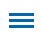The ability to decode and solve word problems is ubiquitous across all state's math standards K-5, but many students struggle to decode the numbers and relationships in these problems.

Visualizing word problems in Wixie by using the paint tools to draw models or by adding images from the media library can help students better identify key pieces of a problem and the relations between them. Students can use these same tools to design their own word problems, building a better understanding of the pieces and relationships in the sentences, as they work to create the structure themselves.

## Recording Explanations

Modern approaches to mathematics learning have moved away from memorization of procedures to strong foundations in number sense and deep conceptual understanding. Wixie's recording tool makes it easy for students to express and explain their mathematical thinking and reasoning during, or as a reflection of, problem-solving.

Few students are interested in written response and audio recording allows them to ramble a bit as they collect and organize their thoughts. This is especially powerful in the primary grades where students are still emerging as writers and even in intermediate grades where they are just learning to type.

These explanations of mathematical thinking help educators evaluate student understanding, or misconceptions, in ways that written student answers to problems may not reveal. These artifacts of learning provide a window into student learning for IEPs and can be helpful in discussions with parents.

Word problems at the primary grades focus on addition and subtraction, with complexity increasing by grade level. For example, in Kindergarten word problems focus on addition and subtraction to ten (CCSS.MATH.CONTENT.K.OA.A.2. First grade progresses to 20 (CCSS.MATH.CONTENT.1.OA.A.1), and in 2nd grade, students are introduced to both one- and two-step word problems (CCSS.MATH.CONTENT.2.OA.A.1).

Because reading and writing are still emerging as skills, keep the focus on mathematical thinking by combining visuals with voice recorded explanations. In this sample, students visualized a math situation and recorded narration to explain the context or problem.

You can also ask students to use the text or paint tools to practice writing number sentences such as 3 + 2 = 5.

If students are beginning to gain confidence in their writing, have them use the Text tool to write sentences. Don't worry about inventive spelling or grammar to keep the focus on mathematical thinking.

Word problems at this level focus on multiplication and division (CCSS.MATH.CONTENT.3.OA.A.3) and being able to tell the difference between additive and multiplicative comparisons (CCSS.MATH.CONTENT.4.OA.A.2).

It is helpful to begin with real world images for visualization purposes, but you will also want to help students learn to use bar models and double number lines to represent the amounts and rates in the word problems they encounter.Explore a Creative Educator lesson plan for this type of word problem project.

If students have devices they can use to capture images, ask them to take pictures of multiplication problems they "see" in the world around them. Ask them to capture and write about these situations and arrays as word problems in a multiplication scrapbook.

In fifth grade, word problem standards focus on fractions (CCSS.MATH.CONTENT.5.NF.A.2) and in sixth grade on ratio, rate, and proportion (CCSS.MATH.CONTENT.6.RPA.A.3).

Wixie includes several word problem activities in the curriculum library at the Activities tab for both fractions and ratio.This is page 2 of a multi-page activity that models how to solve the problem, then presents similar problems with a range of visuals and models (like fraction bars), and concludes with a blank page for students to write their own.

## Connecting Math to the World Beyond the Classroom

To help them build skills and understanding of the contexts that may be found in word problems they encounter on standardized tests, ask students to create posters and trading cards that demonstrate an understanding of the context of how fractions are used in the real world.A fun way to get young students thinking about these connections is with Math Curse by Jon Scieszka and Lane Smith. After reading this fun story about a student who starts to see everything in his world as a math problem, have students use Wixie to write and illustrate a page in your class's own Math Curse story. Have them print and exchange their pages with another student to both edit and solve each other's "problems."

Whether they are using images or bar models, Wixie provides a digital canvas students can use to visualize word problems and create their own to better understand context and structure of the word problems they will encounter in math assessments.

Topics: Wixie, math